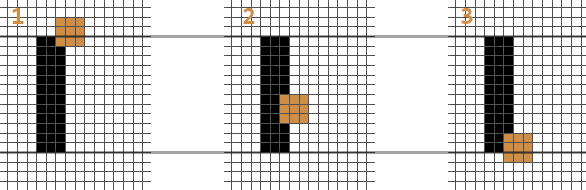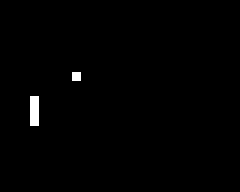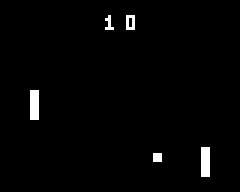# Pong

##### Step 3

The famous game where you hit on the ball with a paddle

• Advanced collision
• Practice all you just learnt

Challenge your friends with a 2 player game. You will build upon everything we have seen until now, as well as expanding your knowledge on ** if** statements.

## Pong, a classic video game

Length 30-40 minutes

**Level **Complete beginner

Prerequisites

Pong, released in 1972, was one of the first arcade video games that became successful. This classic is both fun and easy enough to learn code.

In this workshop, we will recreate this classic. To do so, we will have to use what we saw in the previous workshop to move the ball and control the paddles based on what we did in the tally counter workshop. Let's start with a detailed breakdown of the game as a whole.

## The structure of the game

I have split the game into multiple steps. This allows us to focus on a single smaller task at a time. Trying to figure out the logic behind a game while viewing the game as a whole is... well intimidating and tough. By breaking it down, we will see that Pong, just like any other game, is composed of many simple parts.

• Create the ball and a paddle
• Draw the ball and the paddle
• Update the paddle's position
• Update the ball's position
• Check for collisions between the ball and walls
• Check for collisions between the ball and the paddle
• Check to see if the game is not over
• Add a second paddle
• Count and display the scores

So we will start by creating a 1-player Pong. Once we get that working, adding another player will then be a simple task.

## Implementing a ball and a paddle

``````#include <Gamebuino-Meta.h>

// Ball attributes
int ball_posX = 20;
int ball_posY = 20;
int ball_speedX = 1;
int ball_speedY = 1;
int ball_size = 4;

// paddle1 attributes
int paddle1_posX = 10;
int paddle1_posY = 30;

// Dimensions of both paddles
int paddle_height = 12;
int paddle_width = 3;

void setup() {
gb.begin();
}

void loop() {
while (!gb.update());
gb.display.clear();
}
``````

Here we created the variables necessary to describe two things: the ball and the first paddle. The ball has 2 ints to keep track of its position along the two axes X and Y. For each of those axes, we also have a speed that describes the direction of our ball. The paddle also has an X and Y position. However, having a speed variable is unnecessary because we want to control it with the arrows.`paddle_height` and `paddle_width` are the paddle's dimensions. We also describe the ball's size, but since it is a square, only one variable (`ball_size`) is necessary to memorize both its height and width.

``````void loop() {
while (gb.update());
gb.display.clear();

// Draw the ball
gb.display.fillRect(ball_posX, ball_posY, ball_size, ball_size);
// Draw paddle1
gb.display.fillRect(paddle1_posX, paddle1_posY, paddle_width, paddle_height);
}
``````

Here nothing too new, we are displaying the ball and paddle as rectangle. As a refresher, here is the syntax for `fillRect`:

`gb.display.fillRect( x coordinate of our rectangle , y coordinate of our rectangle , width , height );`

## Controlling the paddle

``````void loop() {

while (gb.update());
gb.display.clear();

// paddle1 movement
if (gb.buttons.repeat(BUTTON_UP, 0)) {
paddle1_posY = paddle1_posY - 1;
}
if (gb.buttons.repeat(BUTTON_DOWN, 0)) {
paddle1_posY = paddle1_posY + 1;
}

// Draw the ball and paddle //
}
``````

This piece of code is what allows us to control the paddle's movement. In preceding workshops, we used the function `gb.buttons.pressed(BUTTON_A)` to check when the user pressed down on button A. But here, we want to move the paddle not WHEN the button was pressed, but rather AS LONG AS the button is pressed. Thankfully, the Gamebuino library offers 5 different functions tied to button behavior:

``````* gb.buttons.pressed()
* gb.buttons.released()
* gb.buttons.held()
* gb.buttons.repeat()
* gb.buttons.timeHeld()
``````

We already know `gb.buttons.pressed()` and we will talk about`gb.buttons.repeat()` shortly. To learn more about what the other 3 functions do, head over to the reference page

`gb.buttons.repeat(BUTTON_UP, 3)` is true every 3 frames while the UP arrow is held down. By putting a 0 where the 3 is, this function is true for every frame where the arrow is held down.

## Collisions

``````void setup() {
// setup
}

void loop() {
// paddle1 movement //

ball_posX = ball_posX + ball_speedX;
ball_posY = ball_posY + ball_speedY;

if (ball_posY < 0) {  // Bounce on top edge
ball_speedY = 1;
}

if (ball_posY > gb.display.height() - ball_size) {  // Bounce on bottom edge
ball_speedY = -1;
}

if (ball_posX > gb.display.width() - ball_size) {  // Bounce on the right edge
ball_speedX = -1;
}

// Draw the ball and paddle //
}
``````

Here we apply everything we have seen previously. We update the ball's position, then we check to see if it collided with the edges of the screen and make it bounce. The only difference is that we only check for three edges: top, bottom, and right. The left side must be "defended" by our paddle. Later, when we will have a second player, we will remove the right wall as well.

Now, if you play the game as it is (go ahead, don't be afraid to try), the ball and paddle move as planed. But the ball goes straight through the paddle! Not very fun right? So let's look into that right now!Unlike the collisions with walls, the paddle moves. We have to check multiple conditions to be sure the ball hits the paddle. Let's take a look at the diagrams above. The black rectangle is the paddle, and the three squares are the three possible positions for the ball. In each situation, we want the ball to bounce off the paddle. There are three conditions to deduce from these diagrams.

First of all, you may have guessed that we only want the ball to bounce when it hits the paddle. When looking at it from the X-axis' point of view, this mean that the left side of the ball is overlapping the right side of the paddle.

Also, we want the ball to bounce even if it is only touching the paddle partially. This corresponds to ball 1 and 3 in the diagrams. In the case of ball 1, we need to check that the bottom of the ball is LOWER than the top of the paddle. In a similar fashion with ball 3, we need the top of the ball to be HIGHER than the bottom of the paddle.

• If the left side of the ball touches the right side of the paddle
• If the bottom of the ball is lower than the top of the paddle
• If the top of the ball is higher than the bottom of the paddle

Complex conditions like this are almost impossible to deduce without some help like the diagram above. I initially made the diagram above on a sheet of paper (I cleaned it up and made it a .png later for this tutorial :P ). This is why you should pretty much always have a pencil and a piece of paper within reach when making games. It really prevents headaches.

So if these three conditions are true, then we change the ball's direction. To implement this, we could place three nested ifs:

``````if (ball_posX == paddle1_posX + paddle_width) {  // If the left side of the ball touches the right side of the paddle
if (ball_posY + ball_size >= paddle1_posY) {  // If the bottom of the ball is lower than the top of the paddle
if (ball_posY <= paddle1_posY + paddle_height) {  // If the top of the ball is higher than the bottom of the paddle
// Bounce off the paddle
}
}

}
``````

However, "nesting" ifs one into another like so creates many brackets, which is not easy to read. In C/C++ there is a way to simplify these "waterfalls" of brackets: conditions can use AND and OR operators. The AND operator is written `&&` and the OR operator is `||`. Here is an example:

``````// If a is 3 AND b is negative
if ((a == 3) && (b < 0)) {
}

// If a is at least 3 OR b is equal to 0
if ((a >= 3) || (b == 0)) {
}
``````

The outermost parentheses `( )` (around all conditions) are required.

For our code, we need to test 3 conditions. But the `&&` and `||` operators are used the same way whether it is with 2, 3, or 12 conditions. Now if you try to place all three of our conditions on the same line, you will have fixed the "waterfall" problem, but created another readability problem: the line is very long. A very long line of code is generally badly seen in the coding community. But we can fix this easily as we are allowed to put line breaks in between conditions (or inside conditions for that matter). Look at the difference:

``````// The three conditions on one line
if ( (ball_posX == paddle1_posX + paddle_width) && (ball_posY + b >= paddle1_posY) && (ball_posY <= paddle1_posY + paddle_height) ) {
// Bounce
}

// The same thing, but with line breaks
if ( (ball_posX == paddle1_posX + paddle_width)
&& (ball_posY + ball_size >= paddle1_posY)
&& (ball_posY <= paddle1_posY + paddle_height) ) {
// Bounce
}
``````

We can now interact with the ball! And when the player misses the ball, it flies off the screen and the game is over. But the player probably wants to play another round. So let's place the ball back into the screen after a game is lost.

``````void loop() {
// Paddle movement //
// Update ball movement + collisions //

if (balle_posX < 0) {
// Reset the ball
int ball_posX = 20;
int ball_posY = 20;
int ball_speedX = 1;
int ball_speedY = 1;
}

// Draw the ball and paddle //
}
``````

Here we go, now when the ball leave the screen on the left side, we put it back on the screen and make it go to the right as not to surprise the player ;) Here is all the code we have written up to this point:``````#include <Gamebuino-Meta.h>
// ball attributes
int ball_posX = 20;
int ball_posY = 20;
int ball_speedX = 1;
int ball_speedY = 1;
int ball_size = 4;

// paddle1 attributes
int paddle1_posX = 10;
int paddle1_posY = 30;

// Dimension of both paddles
int paddle_height = 10;
int paddle_width = 3;

void setup() {
gb.begin();
}

void loop() {
while(!gb.update());
gb.display.clear();

// Update paddle1 position
if (gb.buttons.repeat(BUTTON_UP, 0)) {
paddle1_posY = paddle1_posY - 1;
}
if (gb.buttons.repeat(BUTTON_DOWN, 0)) {
paddle1_posY = paddle1_posY + 1;
}

// Update ball position
ball_posX = ball_posX + ball_speedX;
ball_posY = ball_posY + ball_speedY;

// Collisions with walls
if (ball_posY < 0) {
ball_speedY = 1;
}
if (ball_posY > gb.display.height() - ball_size) {
ball_speedY = -1;
}
if (ball_posX > gb.display.width() - ball_size) {
ball_speedX = -1;
}

// Ball-paddle1 collisions
if ( (ball_posX == paddle1_posX + paddle_width)
&& (ball_posY + ball_size >= paddle1_posY)
&& (ball_posY <= paddle1_posY + paddle_height) ) {
ball_speedX = 1;
}

// Check if the ball left the screen (on the left side)
if (ball_posX < 0) {
// Reset ball
ball_posX = 20;
ball_posY = 20;
ball_speedX = 1;
ball_speedY = 1;
}

// Display ball
gb.display.fillRect(ball_posX, ball_posY, ball_size, ball_size);
// Display paddle1
gb.display.fillRect(paddle1_posX, paddle1_posY, paddle_width, paddle_height);
}
``````

## It's your turn!

We started by breaking down Pong into feasible parts. So far we built the core mechanics of the game. We created and drew a ball and a paddle that both move and interact. If the ball slips by the player (and goes off-screen), we reset the ball. The rest is up to you:

• Add a second paddle
• Use buttons A and B to make it move
• Detect when the ball leaves the right side of the screen
• Count the score of each player
• Draw the score

Tip: To count and draw the score, refresh your memory with the tally counter workshop.

Share your game on social media #gamebuino #pong , we go through them all the time ;)

## Solution example

If you are getting stuck or ran out of ideas, here's what we did :)``````#include <Gamebuino-Meta.h>

// ball attributes
int ball_posX = 20;
int ball_posY = 20;
int ball_speedX = 1;
int ball_speedY = 1;
int ball_size = 4;

// paddle1 attributes
int paddle1_posX = 10;
int paddle1_posY = 30;

// paddle2 attributes
int paddle2_posX = gb.display.width() - 13;
int paddle2_posY = 30;

// Dimension of both paddles
int paddle_height = 10;
int paddle_width = 3;

// Scores
int score1;  // Player 1 score
int score2;  // Player 2 score

void setup() {
gb.begin();
}

void loop() {
while(!gb.update());
gb.display.clear();

// Update paddle positions
if (gb.buttons.repeat(BUTTON_UP, 0)) {
paddle1_posY = paddle1_posY - 1;
}
if (gb.buttons.repeat(BUTTON_DOWN, 0)) {
paddle1_posY = paddle1_posY + 1;
}
if (gb.buttons.repeat(BUTTON_B, 0)) {
paddle2_posY = paddle2_posY - 1;
}
if (gb.buttons.repeat(BUTTON_A, 0)) {
paddle2_posY = paddle2_posY + 1;
}

// Update ball position
ball_posX = ball_posX + ball_speedX;
ball_posY = ball_posY + ball_speedY;

// Collisions with walls
if (ball_posY < 0) {
ball_speedY = 1;
}
if (ball_posY > gb.display.height() - ball_size) {
ball_speedY = -1;
}
if (ball_posX > gb.display.width() - ball_size) {
ball_speedX = -1;
}

// Ball-paddle1 collisions
if ( (ball_posX == paddle1_posX + paddle_width)
&& (ball_posY + ball_size >= paddle1_posY)
&& (ball_posY <= paddle1_posY + paddle_height) ) {
ball_speedX = 1;
}
// Ball-paddle2 collisions
if ( (ball_posX + ball_size == paddle2_posX)
&& (ball_posY + ball_size >= paddle2_posY)
&& (ball_posY <= paddle2_posY + paddle_height) ) {
ball_speedX = -1;
}

// Check if the ball left the screen
if (ball_posX < 0) {
// Reset ball
ball_posX = 20;
ball_posY = 20;
ball_speedX = 1;
ball_speedY = 1;
// Increment player2's score
score2 = score2 + 1;
}
if (balle_posX > gb.display.width()) {
// Reset ball
ball_posX = 20;
ball_posY = 20;
ball_speedX = 1;
ball_speedY = 1;
// Increment player1's score
score1 = score1 + 1;
}

// Display ball
gb.display.fillRect(ball_posX, ball_posY, ball_size, ball_size);
// Display paddle1
gb.display.fillRect(paddle1_posX, paddle1_posY, paddle_width, paddle_height);
// Display paddle2
gb.display.fillRect(paddle2_posX, paddle2_posY, paddle_width, paddle_height);

// Display scores
gb.display.setCursor(35, 5);
gb.display.print(score1);
gb.display.setCursor(42, 5);
gb.display.print(score2);
}
``````

By Julien Giovinazzo

Any questions / comments / suggestions, be sure to drop a comment down below!